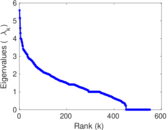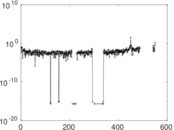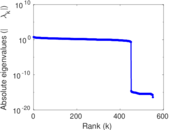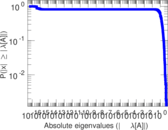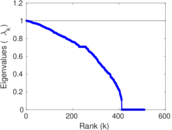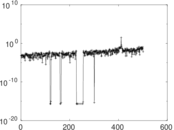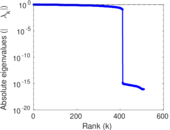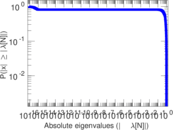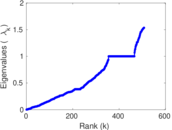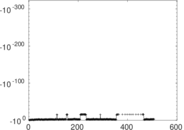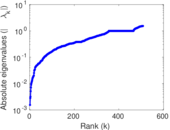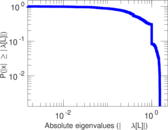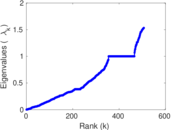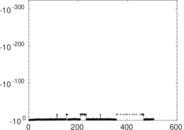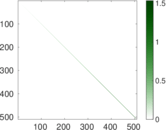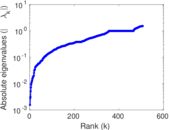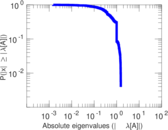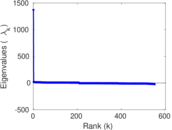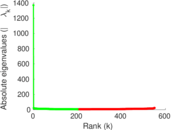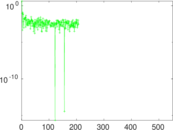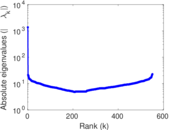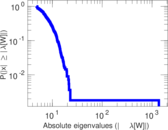# Crime

This bipartite network contains persons who appeared in at least one crime case as either a suspect, a victim, a witness or both a suspect and victim at the same time. A left node represents a person and a right node represents a crime. An edge between two nodes shows that the left node was involved in the crime represented by the right node.

 Code `MC` Internal name `moreno_crime` Name Crime Data source http://moreno.ss.uci.edu/data.html#crime AvailabilityDataset is available for download Consistency checkDataset passed all tests Category Interaction network Node meaning Person, crime Edge meaning Involvment Network formatBipartite, undirected Edge typeUnweighted, no multiple edges

## Statistics

 Size n = 1,380 Left size n1 = 829 Right size n2 = 551 Volume m = 1,476 Wedge count s = 4,816 Claw count z = 12,667 Cross count x = 39,133 Square count q = 143 4-Tour count T4 = 23,424 Maximum degree dmax = 25 Maximum left degree d1max = 25 Maximum right degree d2max = 18 Average degree d = 2.139 13 Average left degree d1 = 1.780 46 Average right degree d2 = 2.678 77 Fill p = 0.003 231 32 Size of LCC N = 1,260 Diameter δ = 32 50-Percentile effective diameter δ0.5 = 12.851 7 90-Percentile effective diameter δ0.9 = 19.474 5 Median distance δM = 13 Mean distance δm = 13.368 7 Gini coefficient G = 0.403 524 Balanced inequality ratio P = 0.349 932 Left balanced inequality ratio P1 = 0.359 079 Right balanced inequality ratio P2 = 0.371 951 Relative edge distribution entropy Her = 0.956 503 Power law exponent γ = 3.008 15 Tail power law exponent γt = 3.901 00 Tail power law exponent with p γ3 = 3.901 00 p-value p = 0.756 000 Left tail power law exponent with p γ3,1 = 2.451 00 Left p-value p1 = 0.137 000 Right tail power law exponent with p γ3,2 = 3.591 00 Right p-value p2 = 0.363 000 Degree assortativity ρ = −0.183 074 Degree assortativity p-value pρ = 1.365 51 × 10−12 Spectral norm α = 5.586 85 Algebraic connectivity a = 0.001 541 74 Spectral separation |λ1[A] / λ2[A]| = 1.082 35 Controllability C = 480 Relative controllability Cr = 0.347 826

## Plots

### Fruchterman–Reingold graph drawing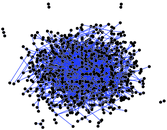### Degree distribution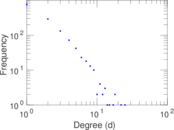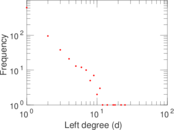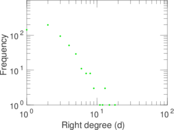### Cumulative degree distribution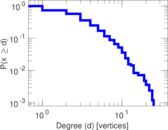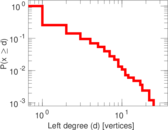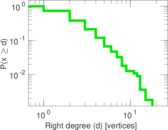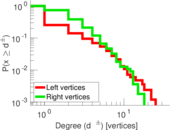### Lorenz curve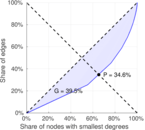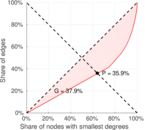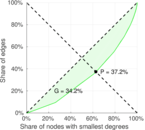### Spectral distribution of the adjacency matrix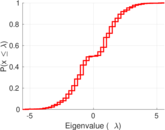### Spectral distribution of the normalized adjacency matrix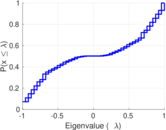### Spectral distribution of the Laplacian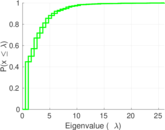### Spectral graph drawing based on the adjacency matrix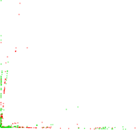### Spectral graph drawing based on the Laplacian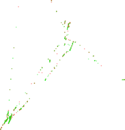### Spectral graph drawing based on the normalized adjacency matrix### Degree assortativity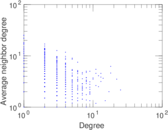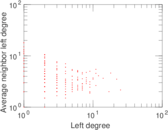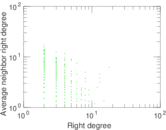### Zipf plot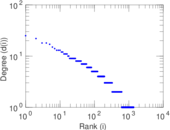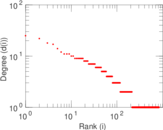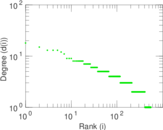### Hop distribution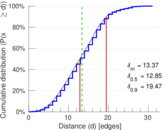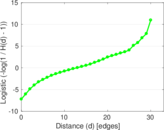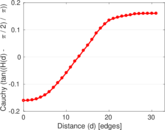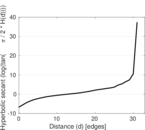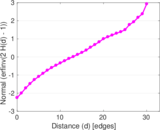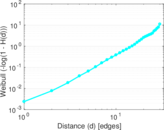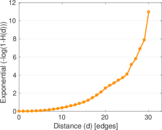### Double Laplacian graph drawing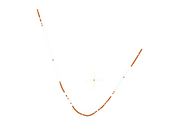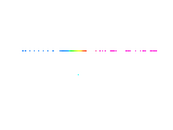### Delaunay graph drawing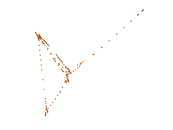### Matrix decompositions plots